• +91 9971497814
• info@interviewmaterial.com

# Chapter 6- Work Energy and power Interview Questions Answers

### Related Subjects

Question 1 :

The sign of work done by a force on a body isimportant to understand. State carefully if the following quantities arepositive or negative:
(a) Work done by a man in liftinga bucket out of a well by means of a rope tied to the bucket,
(b) Work done by gravitationalforce in the above case,
(c) Work done by friction on abody sliding down an inclined plane,
(d) Work done by an applied forceon a body moving on a rough horizontal plane with uniform velocity,
(e) Work done by the resistiveforce of air on a vibrating pendulum in bringing it to rest.

Work done, W = T.S = Fs cos θ
(a) Work done ‘positive’, because force is acting in the direction ofdisplacement i.e., θ = 0°.
(b) Work done is negative, because force is acting against the displacementi.e., θ = 180°.
(c) Work done is negative, because force of friction is acting against thedisplacement i.e., θ= 180°.
(d) Work done is positive, because body moves in the direction of applied forcei.e., θ= 0°.
(e) Work done is negative, because the resistive force of air opposes themotion i.e., θ = 180°.

Question 2 :

A body of mass 2 kg initially at rest movesunder the action of an applied horizontal force of 7 N on a table withcoefficient of kinetic friction = 0.1. Compute the
(a) Work done by the appliedforce in 10 s
(b) Work done by friction in 10 s
(c) Work done by the net force onthe body in 10 s
(d) Change in kinetic energy ofthe body in 10 s and interpret your results.

(a) We know that Uk =frictional force/normal reaction
frictional force = Uk xnormal reaction
= 0.1 x 2 kg wt = 0.1 x 2 x 9.8 N = 1.96 N
net effective force = (7 – 1.96) N = 5.04 N
acceleration = 5.04/2 ms-2 =2.52 ms-2
distance, s=1/2x 2.52 x 10 x 10 = 126 m
work done by applied force = 7 x 126 J = 882 J
(b) Work done by friction = 1.96 x 126 = -246.96 J
(c) Work done by net force = 5.04 x 126 = 635.04 J
(d) Change in the kinetic energy of the body
= work done by the net force in 10 seconds = 635.04 J (This is in accordancewith work-energy theorem).

Question 3 : Given figures are examples of some potential energyfunctions in one dimension. The total energy of the particle is indicated by across on the ordinate axis. In each case, specify the regions, if any, in whichthe particle cannot be found for the given energy. Also, indicate the minimumtotal energy the particle must have in each case. Think of some physicalcontexts for which these potential energy shapes are relevant.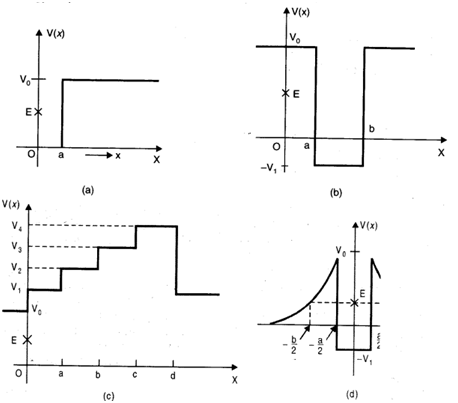We know that total energy E = K.E. + P.E. or K.E. = E – P.E. andkinetic energy can never be negative.The object can not exist in the region,where its K.E. would become negative.
(a) In the region between x = 0 and x = a, potential energy is zero. So,kinetic energy is positive. In the region x > a, the potential energy has avalue greater than E. So, kinetic energy will be negative in this region. Thusthe particle cannot be present in the region x > a.
The minimum total energy that the particle can have in this case is zero.
(b) Here P.E. > E, the total energy of the object and as such the kineticenergy of the object would be negative. Thus object cannot be present in anyregion of the graph.
(c) Here x = 0 to x = a and x > b, the P.E. is more than E, so K.E. isnegative. The particle can not exist in these portions.
(d) The object can not exist in the region between x = -b/2 to x =-a/2 and x =-a/2 to x = -b/2 .Because in this region P.E. > E.

Question 4 : The potential energy function for a particleexecuting linear simple harmonic motion is given by V (x) – kx2/2, where k is the force constant of theoscillator. For k = 0.5 Nm-1 ,the graph of V (x) versus x is shown in Fig. Show that a particle of totalenergy 1 J moving under this potential must ‘turn back’ when it reaches x = ± 2m.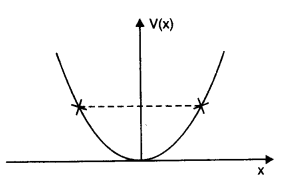Answer 4 : Here, force constant k = 0.5 Nm-1 andtotal energy of particle E = 1J. The particle can go up to a maximum distance xm, where its total energyis transformed into elastic potential energy.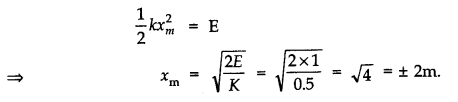(a) The casing of a rocket inflight bums up due to friction. At whose expense is the heat energy requiredfor burning obtained? The rocket or the atmosphere?
(b) Comets move around the sun inhighly elliptical orbits. The gravitational force on the comet due to the sunis not normal to the comet’s velocity in general. Yet the work done by thegravitational force over every complete orbit of the comet is zero. Why?
(c) An artificial satelliteorbiting the earth in very thin atomosphere loses its energy gradually due todissipation against atmospheric resistance, however small. Why then does itsspeed increase progressively as it comes closer and closer to the earth?
(d) In Fig.(i), the man walks 2 mcarrying a mass of 15 kg on his hands. In Fig. (ii), he walks the same distancepulling the rope behind him. The rope goes over a pulley, and a mass of 15 kghangs at its other end. In which case is the work done greater?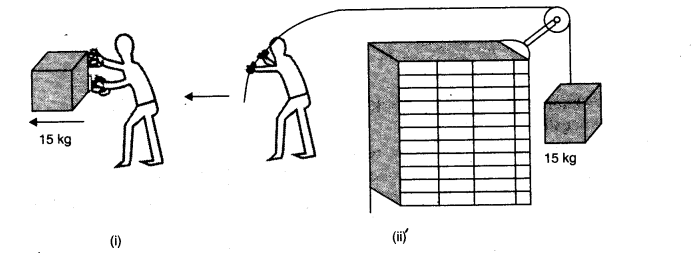Answer 5 : (a) Heat energy required for burning of casing ofrocket comes from the rocket itself. As
a result of work done against friction the kinetic energy of rocketcontinuously decreases – and this work against friction reappears as heatenergy.
(b) This is because gravitational force is a conservative force. Work done bythe gravitational ‘ force of the sun over a closed path in every complete orbitof the comet is zero.
(c)  As an artificial satellite gradually loses its energy due todissipation against atmospheric resistance, its potential decreases rapidly. Asa result, kinetic energy of
satellite slightly increases i.e., its speed increases progressively.
(d) In Fig. (i), force is applied on the mass, by the man in vertically upwarddirection but distance is moved along the horizontal.
θ = 90°. W = Fs cos90° = zero
In Fig. (ii), force is applied along the horizontal and the distance moved isalso along the horizontal. Therefore, θ = 0°.
W = Fs cos θ =mg x s cos 0°
W = 15 x 9.8 x 2 x 1 = 294 joule.
Thus, work done in (ii) case is greater.

Question 6 :  Point out thecorrect alternative:
(a) When a conservative forcedoes positive work on a body, the potential energy of the body increases/decreases/remainsunaltered.
(b)Work done by a body againstfriction always results in a loss of its kinetic/potential energy.
(c) The rate of change of totalmomentum of a many-particle system is proportional to the external force/sum ofthe internal forces of the system.
(d) In an inelastic collision oftwo bodies, the quantities which do not change after the collision are thetotal kinetic energy/total linear momentum/total energy of the system of twobodies.

(a) Potential energy of the body decreases because the body inthis case goes closer to the centre of the force.
(b) Kinetic energy, because friction does its work against the motion.
(c) Internal forces can not change the total or net momentum of a system. Hencethe rate of change of total momentum of many particle system is proportional tothe external force on the system.
(d) In an inelastic collision of two bodies, the quantities which do not changeafter the collision are the total kinetic energy/total linear momentum/ totalenergy of the system of two bodies.

Question 7 :

State if each of the following statements istrue or false. Give reasons for your answer.
(a) In an elastic collision oftwo bodies, the momentum and energy of each body is conserved.
(b) Total energy of a system isalways conserved, no matter what internal and external forces on the bodyare present.
(c) Work done in the motion of abody over a closed loop is zero for every force in nature.
(d) In an inelastic collision,the final kinetic energy is always less than the initial kinetic energy of thesystem.

(a) False, the total momentum and total energy of the system areconserved.
(b) False, the external force on the system may increase or decrease the totalenergy of the system.
(c) False, the work done during the motion of a body over a closed loop is zeroonly when body is moving under the action of a conservative force (such asgravitational or electrostatic force). Friction is not a conservative forcehence work done by force of friction (or work done on the body againstfriction) is not zero over a closed loop.
(d) True, usually in an inelastic collision the final kinetic energy is alwaysless than the initial kinetic energy of the system.

Question 8 :

(a) In an elastic collision oftwo billiard balls, is the total kinetic energy conserved during the short timeof collision of the balls (i.e., when they are in contact)?
(b) Is the total linear momentumconserved during the short time of an elastic collision of two balls?
(c) What are the answers to (a)and (b) for an inelastic collision?
(d) If the potential energy oftwo billiard balls depends only on the separation distance between theircentres, is the collision elastic or inelastic? (Note, we are talking here of potentialenergy corresponding to the force during collision, not gravitational potentialenergy).

(a) In this case total kinetic energy is not conservedbecause when the bodies are in contact dining elastic collision even, thekinetic energy is converted into potential energy.
(b) Yes, because total momentum conserves as per law of conservation ofmomentum.
(d) It is a case of elastic collision because in this case the forces will beof conservative nature.

Question 9 :

A body is initiallyat rest. It undergoes a one-dimensional motion with constant acceleration. Thepower delivered to it at time t is proportional to
(i) t1/2 (ii)t (iii) t3/2 (iv) t2

(ii)From v = u + at
v = 0 + at = at
As power, p = F x  v
.’. p = (ma) x at = ma2t
Since m and a are constants, therefore, p α t.

Question 10 : A body is moving unidirectionally under theinfluence of a source of constant power. Its displacement in time t isproportional to (i) t1/2 (ii)t (iii) t3/2 (iv) t2

Answer 10 : (ii)p = force x velocity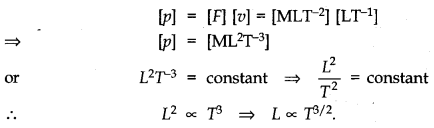Todays Deals### Chapter 6- Work Energy and power Contributorskrishan

Name:
Email:

# Latest News# 9000 interview questions in different categories